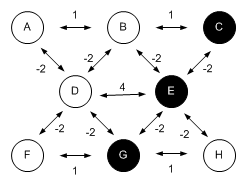### CSC 550: Introduction to Artificial Intelligence Fall 2008 HW5: Neural Nets

1. In class, we trained a backpropagation network to predict a person's political affiliation based on survey data. For this assignment, you are to similarly train a backpropagation network using the data you obtained for Homework 4. Recall that you had to obtain a data collection and use the ID3 algorithm to build a decision tree for classifying samples from that collection. Now, you are to adapt that data to a neural network model, using the backpropogation applet we experimented with in class.

You will need to select a subset of samples from your data collection and use them to train a network. Then, test the resulting network on remaining samples and see how well it does in classifying the results. Provide a screenshot or drawing of your trained network and commentary on its effectivess.

2. Consider the following Hopfield net, where active nodes are drawn as shaded circles while inactive nodes are not shaded.1. Starting from this state (with active nodes C, E, and G), identify a sequence of states obtained by performing parallel relaxation. Reminder: a node should become active when the sum of the weights from its active neighbors exceeds 0, and should likewise become inactive when the sum is below 0. Parallel relaxation repeatedly finds an invalid node (either an inactive node that should be active, or vice versa) and toggles it. The process terminates when there are no invalid nodes in the net, i.e., it has reached a stable state.

2. Is there more than one stable state reachable from this start state? If so, which one is "closest" to the start state (i.e., differs by the fewest nodes). Justify your answer.

3. Identify all of the stable states of this particular Hopfield network? How did you determine this?

4. Implement this Hopfield net as a Scheme structure (similar to the Hopfield net defined in hopfieldBFS.scm). Verify your answers to the above questions using the relax function defined in that same file.